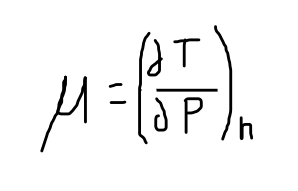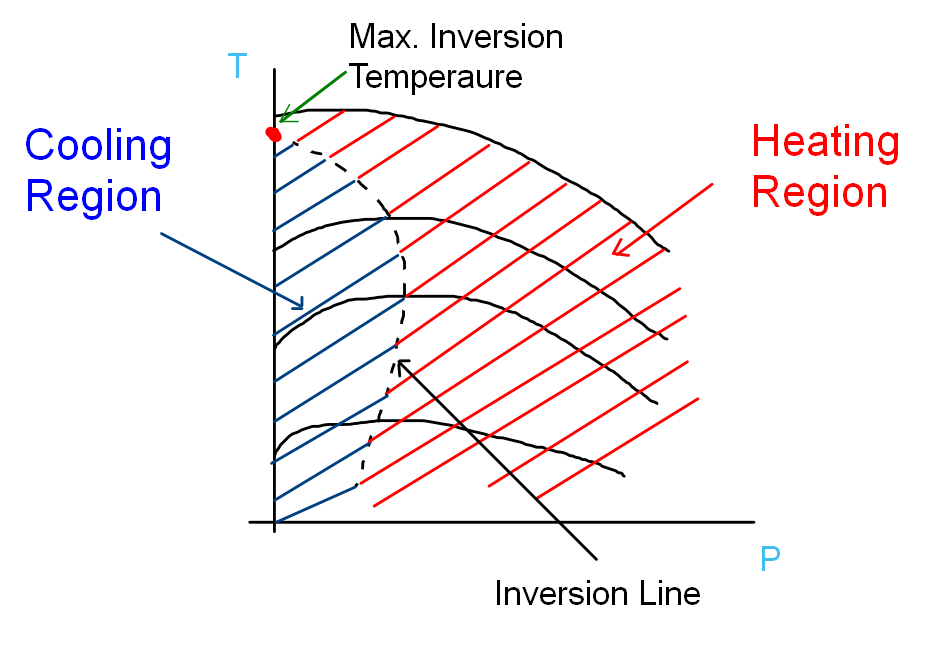top of page

# Throttling Process

Updated: Nov 5

The expansion of the fluid in the expansion valve/turbines results in a pressure drop in the thermal systems which produces thermodynamic work. Sometimes there is a need to reduce the fluid pressure without producing any thermodynamic work. This pressure drop is obtained by using throttling valves, which result in the pressure drop and do not produce any thermodynamic work.

What is the throttling process?

Throttling is a process of reducing the pressure of the fluid significantly by passing it through flow-restricting devices.

Which devices are used in throttling?

An Orifice, capillary tube, porous plugs, etcThrottling Devices

Does the throttling process produce any thermodynamic work?

No. Throttling does not produce any thermodynamic work.

Which property remains constant during the throttling process?

Enthalpy. That is why the throttling process is called an isenthalpic process.

Why does enthalpy remain constant during the isenthalpic process?

Let's apply the energy balance equation between the upstream and downstream of the throttling valve.

Q + h1= W + h2 + d(KE) + d(PE)

Q = 0

Reason: There is not enough time and surface area available for heat transfer

W = 0

Reason: There is no lifting of any weight (no thermodynamic work)

d(PE) = 0

Reason: There is no potential head difference

d(KE) =~ 0

Reason: there may be an increase in velocity at the outlet compared to the inlet but in many cases the change in kinetic energy is insignificant.

Hence we remain with

###### h1=~h2 (kJ/Kg)

i.e.

u1 + P1v1 = u2 + P2v2

internal energy + flow work = constant

What happens to temperature in the throttling process? Does internal energy change in throttling?

For throttling processes,

internal energy + flow work = constant

If we talk in terms of internal energy and flow work, If flow energy increases during the process it causes the internal energy to decrease. Temperature is directly dependent on internal energy hence in this case the temperature reduces. On the other hand, if the flow energy reduces downstream (the work required to push fluid is less at the outlet) and internal energy rises the temperature of the fluid increases.

The magnitude of rise or drop in temperature is decided by the Joule-Thomson coefficient.

What is Joule-Thomson coefficient?

It is a measure of the rate of change of temperature with respect to pressure during a constant enthalpy process.Remember that in the throttling process the pressure always drops. So, the denominator is always negative in the equation. Hence, if the temperature of the fluid drops the Joule-Thomson coefficient is positive. Conversely, if the temperature of the fluid increases Joule-Thomson coefficient is negative.

Now, let's understand T-P plot with a single constant enthalpy line as shown below.Constant Enthalpy Line

This constant enthalpy line is drawn by doing experiments using different sizes of porous plugs and different initial pressure and temperature conditions. The outlet pressure and temperature are plotted for each inlet condition of temperature and pressure with different sizes of porous plugs.Constant enthalpy lines on T-P Plot with different initial conditions and different sizes of throttling devices

Each constant enthalpy line on the T-P plot has one zero slope point (where the Joule-Thomson coefficient is zero).

What is the inversion line?

The locus of all the points at which the Joule-Thomson coefficient is zero is called the inversion line or inversion curve.Inversion Line and Maximum Inversion Temperature

What is inversion temperature?

The temperature at a point where the constant enthalpy line intersects the inversion line is called inversion temperature.

What is the maximum inversion temperature?

The point at which the inversion curve intersects the y axis (zero pressure line) is the maximum inversion temperature.

If we look closely the slope of isenthalpic lines is negative to the right of the inversion curve which is nothing but the heating region and the slope is positive to the right of the inversion curve which is the cooling region. So, if the throttling is done in the heating region the temperature of the fluid increases whereas, the temperature drops if the throttling starts to the left of the inversion curve.Inversion Line and Heating/Cooling Regions

If the cooling effect is needed after throttling then the initial temperature of the fluid must be below the maximum inversion temperature.

For almost all the fluids this holds true naturally because their maximum inversion temperature is above room temperature. The only exceptions are Helium and Hydrogen where the maximum inversion temperatures are very low. Hence if we throttle hydrogen or helium at room temperatures they heat up even after throttling.

Does the throttling valve increase velocity?

Generally, the velocity at the downstream of throttling valve is more than the inlet velocity but the effect of change in velocity(KE) is insignificant in the energy balance equation.

What is constant in the throttling process? Is the throttling process an adiabatic process?

Enthalpy is constant in the throttling process that is why it is called the isenthalpic process. The heat transfer is negligible in the throttling process because it happens in a very short period of time as well as the surface area available for heat transfer is low. But capillary tubes can be the exception to this because they provide a higher surface area for heat transfer.

Why throttling process is highly irreversible?

The flow accelerates through a small passage but the flow then separates at the downstream of throttling valve. The high-speed fluid mixes with the low-speed fluid and all that shearing and roiling is what causes the dissipation. Friction is the number one reason for all the thermodynamic irreversibilities.

Why temperature does not drop when ideal gas is throttled?

Enthalpy of an ideal gas is a function of temperature only. h=h(T), which requires that temperature remains constant when the enthalpy remains constant. Therefore, the throttling process can not be used to lower the temperature of an ideal gas.

What is the application of the throttling process in thermal systems?

The throttling process is used in the vapor compression refrigeration systems because they reduce the pressure of the high-pressure refrigerant liquid which came out of the condenser to lower pressure. This causes a significant drop in the temperature of the refrigerant. This low-temperature refrigerant liquid-vapor mixture is then used to absorb heat from the refrigeration space. The advantage of using a throttling valve is that it does not produce any thermodynamic work and throttling valves are very cost-effective compared to expansion devices such as turbines. Hence, they cause a significant reduction in the initial cost of VCRS.